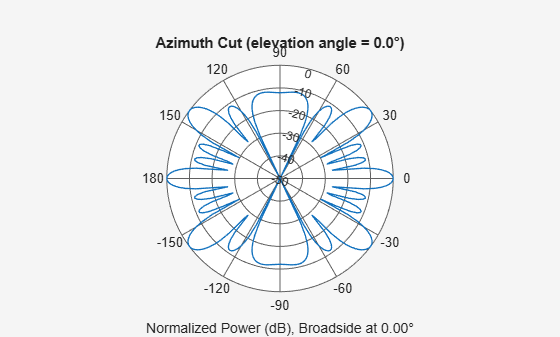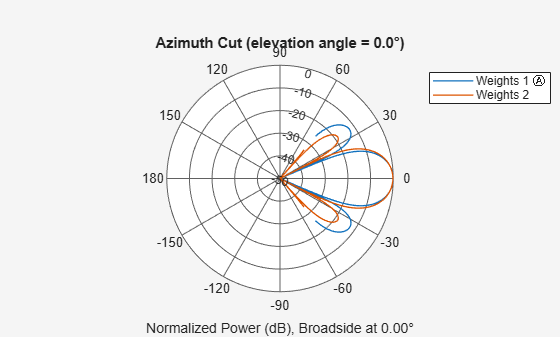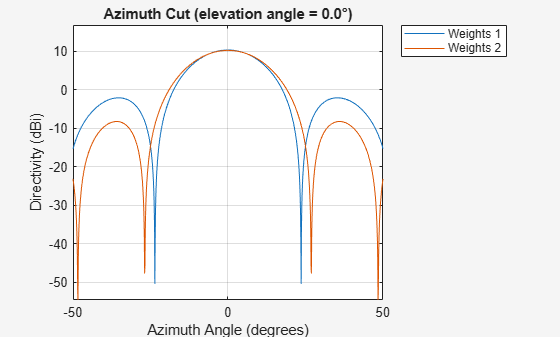# pattern

System object: phased.PartitionedArray
Package: phased

Plot partitioned array directivity, field, and power patterns

## Syntax

```pattern(sArray,FREQ) pattern(sArray,FREQ,AZ) pattern(sArray,FREQ,AZ,EL) pattern(___,Name,Value) [PAT,AZ_ANG,EL_ANG] = pattern(___) ```

## Description

`pattern(sArray,FREQ)` plots the 3-D array directivity pattern (in dBi) for the array specified in `sArray`. The operating frequency is specified in `FREQ`.

The integration used when computing array directivity has a minimum sampling grid of 0.1 degrees. If an array pattern has a beamwidth smaller than this, the directivity value will be inaccurate.

`pattern(sArray,FREQ,AZ)` plots the array directivity pattern at the specified azimuth angle.

`pattern(sArray,FREQ,AZ,EL)` plots the array directivity pattern at specified azimuth and elevation angles.

`pattern(___,Name,Value)` plots the array pattern with additional options specified by one or more `Name,Value` pair arguments.

`[PAT,AZ_ANG,EL_ANG] = pattern(___)` returns the array pattern in `PAT`. The `AZ_ANG` output contains the coordinate values corresponding to the rows of `PAT`. The `EL_ANG` output contains the coordinate values corresponding to the columns of `PAT`. If the `'CoordinateSystem'` parameter is set to `'uv'`, then `AZ_ANG` contains the U coordinates of the pattern and `EL_ANG` contains the V coordinates of the pattern. Otherwise, they are in angular units in degrees. UV units are dimensionless.

### Note

This method replaces the `plotResponse` method. See Convert plotResponse to pattern for guidelines on how to use `pattern` in place of `plotResponse`.

## Input Arguments

expand all

Partitioned array, specified as a `phased.PartitionedArray` System object.

Example: `sArray= phased.PartitionedArray;`

Frequencies for computing directivity and patterns, specified as a positive scalar or 1-by-L real-valued row vector. Frequency units are in hertz.

• For an antenna, microphone, or sonar hydrophone or projector element, `FREQ` must lie within the range of values specified by the `FrequencyRange` or `FrequencyVector` property of the element. Otherwise, the element produces no response and the directivity is returned as `–Inf`. Most elements use the `FrequencyRange` property except for `phased.CustomAntennaElement` and `phased.CustomMicrophoneElement`, which use the `FrequencyVector` property.

• For an array of elements, `FREQ` must lie within the frequency range of the elements that make up the array. Otherwise, the array produces no response and the directivity is returned as `–Inf`.

Example: `[1e8 2e6]`

Data Types: `double`

Azimuth angles for computing directivity and pattern, specified as a 1-by-N real-valued row vector where N is the number of azimuth angles. Angle units are in degrees. Azimuth angles must lie between –180° and 180°.

The azimuth angle is the angle between the x-axis and the projection of the direction vector onto the xy plane. When measured from the x-axis toward the y-axis, this angle is positive.

Example: `[-45:2:45]`

Data Types: `double`

Elevation angles for computing directivity and pattern, specified as a 1-by-M real-valued row vector where M is the number of desired elevation directions. Angle units are in degrees. The elevation angle must lie between –90° and 90°.

The elevation angle is the angle between the direction vector and xy-plane. The elevation angle is positive when measured towards the z-axis.

Example: `[-75:1:70]`

Data Types: `double`

### Name-Value Pair Arguments

Specify optional comma-separated pairs of `Name,Value` arguments. `Name` is the argument name and `Value` is the corresponding value. `Name` must appear inside quotes. You can specify several name and value pair arguments in any order as `Name1,Value1,...,NameN,ValueN`.

Plotting coordinate system of the pattern, specified as the comma-separated pair consisting of `'CoordinateSystem'` and one of `'polar'`, `'rectangular'`, or `'uv'`. When `'CoordinateSystem'` is set to `'polar'` or `'rectangular'`, the `AZ` and `EL` arguments specify the pattern azimuth and elevation, respectively. `AZ` values must lie between –180° and 180°. `EL` values must lie between –90° and 90°. If `'CoordinateSystem'` is set to `'uv'`, `AZ` and `EL` then specify U and V coordinates, respectively. `AZ` and `EL` must lie between -1 and 1.

Example: `'uv'`

Data Types: `char`

Displayed pattern type, specified as the comma-separated pair consisting of `'Type'` and one of

• `'directivity'` — directivity pattern measured in dBi.

• `'efield'` — field pattern of the sensor or array. For acoustic sensors, the displayed pattern is for the scalar sound field.

• `'power'` — power pattern of the sensor or array defined as the square of the field pattern.

• `'powerdb'` — power pattern converted to dB.

Example: `'powerdb'`

Data Types: `char`

Display normalized pattern, specified as the comma-separated pair consisting of `'Normalize`' and a Boolean. Set this parameter to `true` to display a normalized pattern. This parameter does not apply when you set `'Type'` to `'directivity'`. Directivity patterns are already normalized.

Data Types: `logical`

Plotting style, specified as the comma-separated pair consisting of `'Plotstyle'` and either `'overlay'` or `'waterfall'`. This parameter applies when you specify multiple frequencies in `FREQ` in 2-D plots. You can draw 2-D plots by setting one of the arguments `AZ` or `EL` to a scalar.

Data Types: `char`

Polarized field component to display, specified as the comma-separated pair consisting of 'Polarization' and `'combined'`, `'H'`, or `'V'`. This parameter applies only when the sensors are polarization-capable and when the `'Type'` parameter is not set to `'directivity'`. This table shows the meaning of the display options.

`'Polarization'`Display
`'combined'`Combined H and V polarization components
`'H'`H polarization component
`'V'`V polarization component

Example: `'V'`

Data Types: `char`

Signal propagation speed, specified as the comma-separated pair consisting of `'PropagationSpeed'` and a positive scalar in meters per second.

Example: `'PropagationSpeed',physconst('LightSpeed')`

Data Types: `double`

Subarray weights, specified as the comma-separated pair consisting of `'Weights`' and an N-by-1 complex-valued column vector or N-by-M complex-valued matrix. The dimension N is the number of subarrays in the array. The dimension L is the number of frequencies specified by the `FREQ` argument.

`Weights` dimension`FREQ` dimensionPurpose
N-by-1 complex-valued column vectorScalar or 1-by-L row vectorApplies a set of weights for the single frequency or for all L frequencies.
N-by-L complex-valued matrix1-by-L row vectorApplies each of the L columns of `‘Weights’` for the corresponding frequency in the `FREQ` argument.

Example: `'Weights',ones(N,M)`

Data Types: `double`

Subarray steering angle, specified as the comma-separated pair consisting of `'SteerAngle'` and a scalar or a 2-by-1 column vector.

If `'SteerAngle'` is a 2-by-1 column vector, it has the form `[azimuth; elevation]`. The azimuth angle must be between –180° and 180°, inclusive. The elevation angle must be between –90° and 90°, inclusive.

If `'SteerAngle'` is a scalar, it specifies the azimuth angle only. In this case, the elevation angle is assumed to be 0.

This option applies only when the `'SubarraySteering'` property of the System object is set to `'Phase'` or `'Time'`.

Example: `'SteerAngle',[20;30]`

Data Types: `double`

Subarray element weights, specified as complex-valued NSE-by-N matrix or 1-by-N cell array. Weights are applied to the individual elements within a subarray. Subarrays can have different dimensions and sizes.

If `ElementWeights` is a complex-valued NSE-by-N matrix, NSE is the number of elements in the largest subarray and N is the number of subarrays. Each column of the matrix specifies the weights for the corresponding subarray. Only the first K entries in each column are applied as weights where K is the number of elements in the corresponding subarray.

If `ElementWeights` is a 1-by-N cell array. Each cell contains a complex-valued column vector of weights for the corresponding subarray. The column vectors have lengths equal to the number of elements in the corresponding subarray.

#### Dependencies

To enable this name-value pair, set the `SubarraySteering` property of the array to `'Custom'`.

Data Types: `double`
Complex Number Support: Yes

## Output Arguments

expand all

Array pattern, returned as an M-by-N real-valued matrix. The dimensions of `PAT` correspond to the dimensions of the output arguments `AZ_ANG` and `EL_ANG`.

Azimuth angles for displaying directivity or response pattern, returned as a scalar or 1-by-N real-valued row vector corresponding to the dimension set in `AZ`. The columns of `PAT` correspond to the values in `AZ_ANG`. Units are in degrees.

Elevation angles for displaying directivity or response, returned as a scalar or 1-by-M real-valued row vector corresponding to the dimension set in `EL`. The rows of `PAT` correspond to the values in `EL_ANG`. Units are in degrees.

## Examples

expand all

Plot the azimuth response of a 4-element ULA partitioned into two 2-element ULA's. The element spacing is one-half wavelength.

Create the ULA, and partition it into two 2-element ULA's.

```sULA = phased.ULA('NumElements',4,'ElementSpacing',0.5); sPA = phased.PartitionedArray('Array',sULA,... 'SubarraySelection',[1 1 0 0;0 0 1 1]);```

Plot the azimuth response of the array. Assume the operating frequency is 1 GHz and the propagation speed is the speed of light.

```fc = 1e9; pattern(sPA,fc,[-180:180],0,'Type','powerdb',... 'CoordinateSystem','polar',... 'Normalize',true)```Convert a 2-by-6 URA of isotropic antenna elements into a 1-by-3 partitioned array so that each subarray of the partitioned array is a 2-by-2 URA. Assume that the frequency response of the elements lies between 1 and 6 GHz. The elements are spaced one-half wavelength apart corresponding to the highest frequency of the element response. Plot an azimuth cut from -50 to 50 degrees for different two sets of weights. For partitioned arrays, weights are applied to the subarrays instead of the elements.

Create partitioned array

```fmin = 1e9; fmax = 6e9; c = physconst('LightSpeed'); lam = c/fmax; sIso = phased.IsotropicAntennaElement(... 'FrequencyRange',[fmin,fmax],... 'BackBaffled',false); sURA = phased.URA('Element',sIso,'Size',[2,6],... 'ElementSpacing',[lam/2,lam/2]); subarraymap = [[1,1,1,1,0,0,0,0,0,0,0,0];... [0,0,0,0,1,1,1,1,0,0,0,0];... [0,0,0,0,0,0,0,0,1,1,1,1]]; sPA = phased.PartitionedArray('Array',sURA,... 'SubarraySelection',subarraymap);```

Plot power pattern

Plot the response of the array at 5 GHz over the restricted range of azimuth angles.

```fc = 5e9; wts = [[1,1,1]',[.862,1.23,.862]']; pattern(sPA,fc,[-50:0.1:50],0,... 'Type','powerdb',... 'CoordinateSystem','polar',... 'Weights',wts)```The plot of the response shows the broadening of the main lobe and the reduction of the strength of the sidelobes caused by the weight tapering.

Plot directivity

Plot an azimuth cut of the directivity of the array at 5 GHz over the restricted range of azimuth angles for the two different sets of weights.

```fc = 5e9; wts = [[1,1,1]',[.862,1.23,.862]']; pattern(sPA,fc,[-50:0.1:50],0,... 'Type','directivity',... 'CoordinateSystem','rectangular',... 'Weights',wts)```